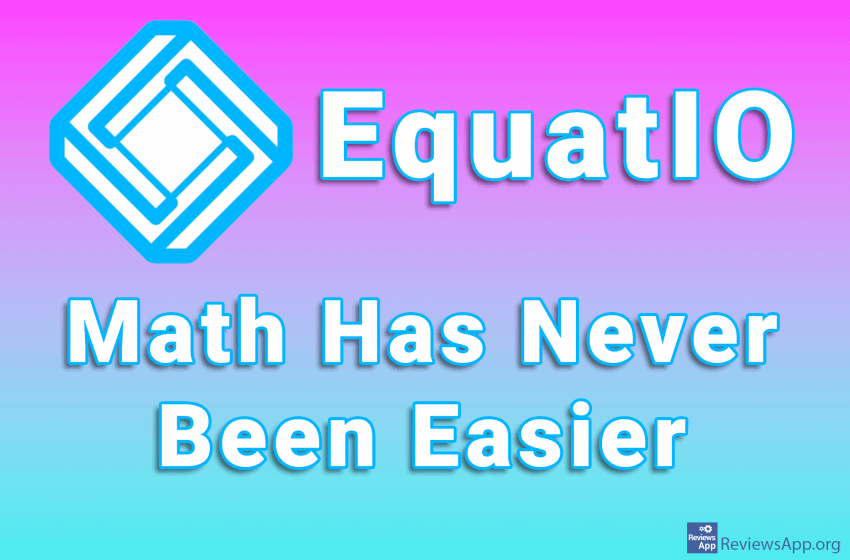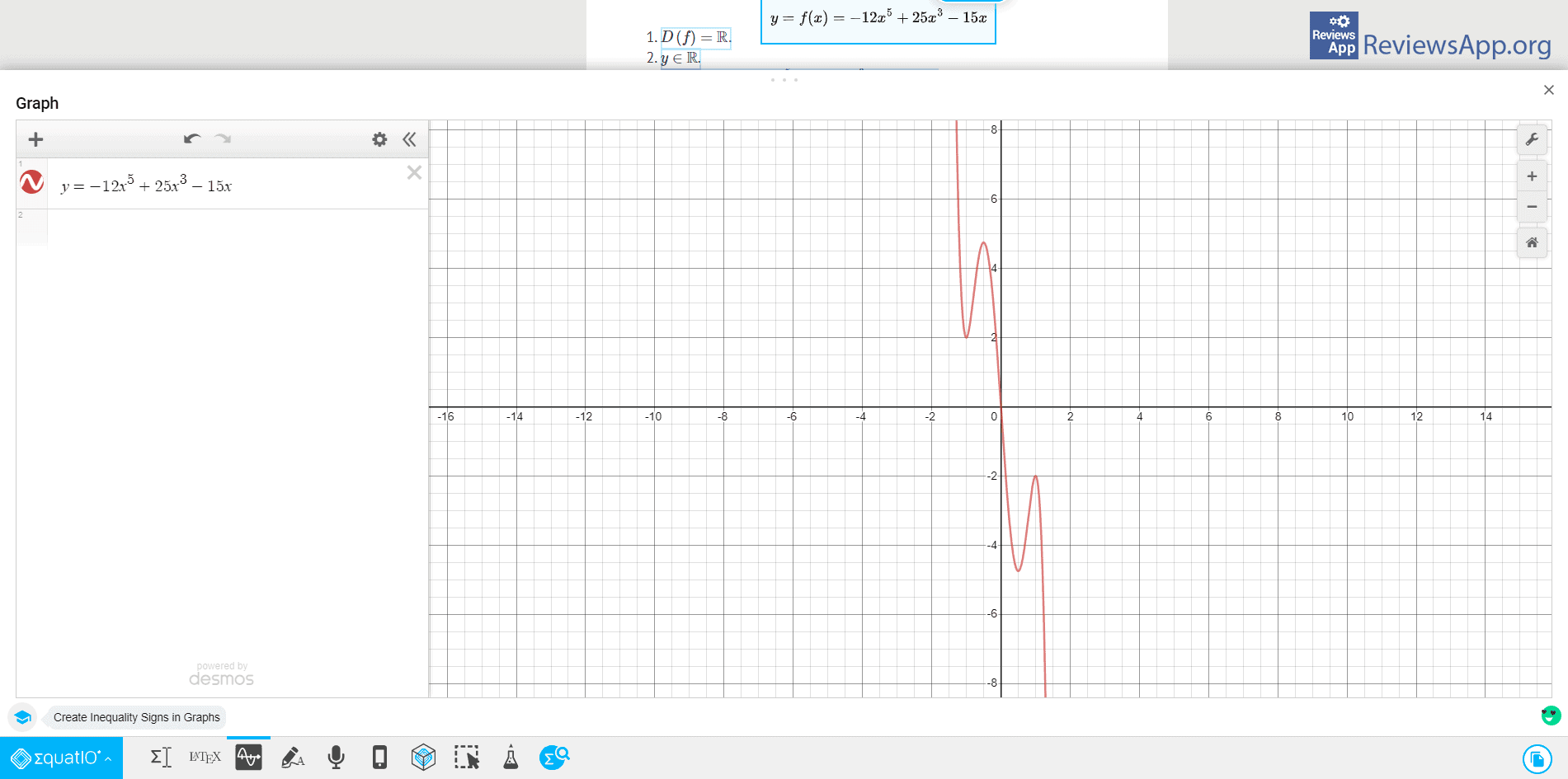# EquatIO – Math Has Never Been EasierAs we all know, math is one of the easiest and most popular subjects in school. Because of that, it is almost unheard of for students to take extra lessons from a subject that is as easy as math. Aids like calculators are completely useless, and we have to ask ourselves why someone wasted time and money making this device.

If you agree with this statement, first of all, good for you, and you have our complete respect and admiration. On the other hand, if you recognize it as a joke, then, like us, you belong to a larger group of people to whom math is one of the hardest things in the world.

Whatever group you belong to, a quality tool for solving mathematical problems is always welcome. Introducing EquatIO, a free program for Windows 7, 8, 10, and macOS, as well as a plugin for Google Chrome.

## How does EquatIO work?

EquatIO is a program for solving mathematical problems, so the question is how to enter the formula into the program. This can be done in three ways. The first is using the keyboard, using the Equation Editor. In addition to the mathematical characters on the keyboard, degrees, roots, fractions, integrals, and all other mathematical operations can be entered.

The second and, in our opinion, the easier way is to write the formula by hand, that is, with the mouse. Writing a formula is like drawing in Paint, and the program is smart enough to recognize what you wrote without error.The third way is to scan the formula written in a text document, image, or web page. If you use the Chrome plugin, there is also an option to detect all mathematical formulas on the website automatically. Then, all you have to do is click on the formula, and it will be entered into the program.

When the mathematical formula is written in the program, the main thing is solving the problem. EquatIO will solve the task for you and show the procedure used to solve the task. If it is a function, a graph will also be displayed on the screen. There are also options to write a note, create a table associated with the function, and copy all this to Word, Excel, and Google Docs.## Conclusion

EquatIO is a phenomenal program. The user interface is modern, clean, and easy to use, and the program works quickly and without errors. It is great for students, teachers, and anyone who likes math or doesn’t like it but is forced to use it at work.

Platform:
Windows 7, 8, 10, macOS, and a plugin for Google Chrome#### Rate:(No Ratings Yet)Loading...